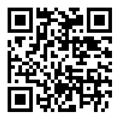1. 中美双边农产品出口三元边际及关税效应分析[J].农业技术经济,2019(03).

2. 基于产销及地域视角的鸡蛋价格空间传导分析[J]. 农业技术经济,202007.

3. 国际农产品价格对国内农产品价格动态传递效应[J]. 国际贸易问题,201908.

4. 交易特性、农户产业组织模式选择与增收效应 ——基于多元Logit模型和MTE模型分析[J].南京农业大学学报（社会科学版）,2019(05).

5. 中国肉羊产业时空演变的特征分析[J].华中农业大学学报(社会科学版),2018(01).

6. 农产品价格支持政策对农产品期现货市场关联影响[J]. 华中农业大学学报(社会科学版),2020(03).

7. 贸易开放背景下国内外羊毛市场关联与溢出效应研究[J].国际商务(对外经济贸易大学学报),2019(05).

8. “一带一路”背景下中俄双边农产品出口三元边际分析[J]. 现代经济探讨,2018(10).

9. 贸易开放视角下国内外羊毛价格动态传递效应分析[J].干旱区资源与环境,201812.

10. 中国与中亚西亚经济走廊国家农产品贸易特征分析——基于“一带一路”战略背景[J].经济问题探索,2018(06).

11. 国内和国际棉花期现货市场溢出效应与动态关联研究——基于不同政策背景下比较分析[J].中南大学学报（社会科学版）,201806.

12. 中国与新亚欧大陆桥沿线国家农产品贸易特征——“一带一路”背景下的分析[J].哈尔滨工业大学学报(社会科学版),2017,19(05).

13.  “一带一路”背景下中国对东南亚国家农产品出口三元边际特征及其影响因素分析[J].当代经济管理,2019(06).

14. 中国与“一带一路”沿线地区农产品产业内贸易分析[J].当代经济管理,2018(11).

15. 我国羊肉供需现状及趋势分析[J].农业经济与管理,2016(03).

16. 我国羊肉价格波动特征及替代品价格冲击效应分析[J].农林经济管理学报,2017,16(03).

17. 交易特性对养殖户产业组织模式选择的影响分析[J].农业经济与管理,2019(02).

18. 禽流感危机下肉鸡产业市场状态分析 ——基于互联网大数据和MS-VAR模型[J].中国农业资源与区划,2018, (12).

19. 中国肉类产量变量特征及因素贡献分解研究[J].世界农业,2017(06).

20. 中国鸡蛋产业链不同市场环节价格传导效应分析[J].农林经济管理学报,2018,17(06).

21. 禽流感疫情对我国畜禽产品价格波动的影响[J].农业经济与管理,2018(02) .

22. 中国农业纺织原料市场价格溢出效应与动态关联研究——基于不同政策背景下比较分析[J].中国农业大学学报,201906.

23. 人民币汇率变动对中国农产品价格的动态传递效应分析——基于TVP-VAR模型[J].中国农业大学学报,201812.

24. 中国畜牧业生产波动的特征及路径识别[J].农业现代化研究,2018,39(02).